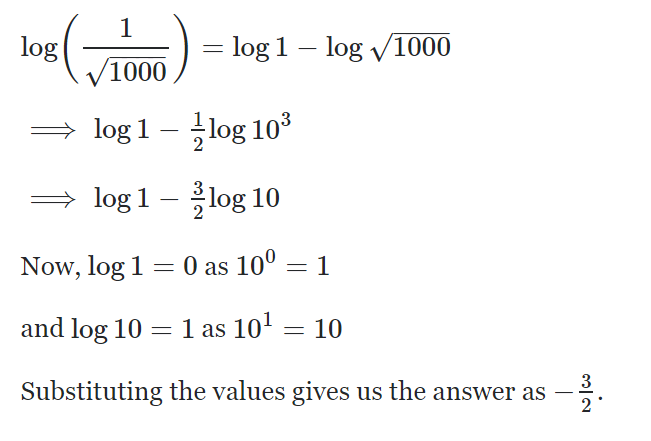# [ Archived Post ] Notes about estimating the True values using likelihood

Please note that this post is for my own educational purpose. Hence I do not describe anything in detail, I am just making notes!

D represent the data that we have observed, and F is the true value of that data. Here we don’t have the true data F, we want to estimate it, for that we are already assuming that the error of the observed data is gaussian. Additionally, we already have estimate the standard deviation of the guassian term, which is represented as an error term. Now we have to note that there are multiple of observed data not just one.

Done, but the likelihood can be a small number, multiply bunch of small number == smaller number. Take the log. Lets review some log rules.Image from this website and this website this website is useful as well

Done, now lets view it in a clear view.

The next step is simple, just take the derivative and set to 0, since we want it to be maximized.

Great, we took the derivative and now we can analytically solve this problem, lets rewrite it more clearly.

Also we can estimate the bound of uncertainty from and that is shown in the right. Now lets actually see this effect in python.

This method does gives us a reasonable estimation with error bound of 4 up and down. Now lets do the Bayesian approach.

Which is the conditional probability of all true value given all of the observed data.

And the simple equation can expanded like above, but we don’t know P(Ftrue) so we would just give a flat probability of 1. And then we can see that the above optimization is similar to the optimization we solved before. (but just divided with P(D), hence they are proportional.)

So using a couple of high level api’s and sampling we are going to sample from posterior probability distribution.

And we get our estimate of the value similar to analytical method we saw above. I will dig more into the MCMC sampling method. But for now lets take a look at higher divisional data. But I want to review some concepts.

P(True | Data) → Posterior, given the data the probability of the model
P(Data | True) → likelihood, given true model probability of observing data
P(True) → model prior
P(Data) → data probability

Now True is not just a single float number it is a distribution, sure the mean (u) and std (sigma) values are a real number but the number of True values are not.

We can already see that the true values are not static number, rather forms a distribution around 1000. And due to this stochasticity the observed data points are also quite in the range.

Taking the analytical approach seems like a good idea, but now the there are two values we need to optimize the sigma and the mean (u) and we only have one equation. Hence taking the derivative respect to each value and setting it to zero means that we have to know the optimal value of another variable before hand.

Using the advance method we can estimate the mean and the variance, we can additionally take the Bayesian method.

The second estimation using the Bayesian method gives a poor results but still very good.

# Maximum Likelihood, clearly explained!!!

Reference

1. Logarithm Rules — ChiliMath. (2018). ChiliMath. Retrieved 13 November 2018, from https://www.chilimath.com/lessons/advanced-algebra/logarithm-rules/
2. (1/\sqrt{1000})$?, H. (2018). How do I simplify$\log (1/\sqrt{1000})\$?. Mathematics Stack Exchange. Retrieved 13 November 2018, from https://math.stackexchange.com/questions/850622/how-do-i-simplify-log-1-sqrt1000
3. StatQuest: Maximum Likelihood, clearly explained!!!. (2018). YouTube. Retrieved 13 November 2018, from https://www.youtube.com/watch?v=XepXtl9YKwc
4. Model, M. (2018). Maximum Likelihood Estimation of Coefficients for Linear Regression Model. Cross Validated. Retrieved 13 November 2018, from https://stats.stackexchange.com/questions/210995/maximum-likelihood-estimation-of-coefficients-for-linear-regression-model
5. VanderPlas, J. (2018). Frequentism and Bayesianism: A Practical Introduction | Pythonic Perambulations. Jakevdp.github.io. Retrieved 13 November 2018, from http://jakevdp.github.io/blog/2014/03/11/frequentism-and-bayesianism-a-practical-intro/

https://jaedukseo.me I love to make my own notes my guy, let's get LIT with KNOWLEDGE in my GARAGE

## More from Jae Duk Seo

https://jaedukseo.me I love to make my own notes my guy, let's get LIT with KNOWLEDGE in my GARAGE